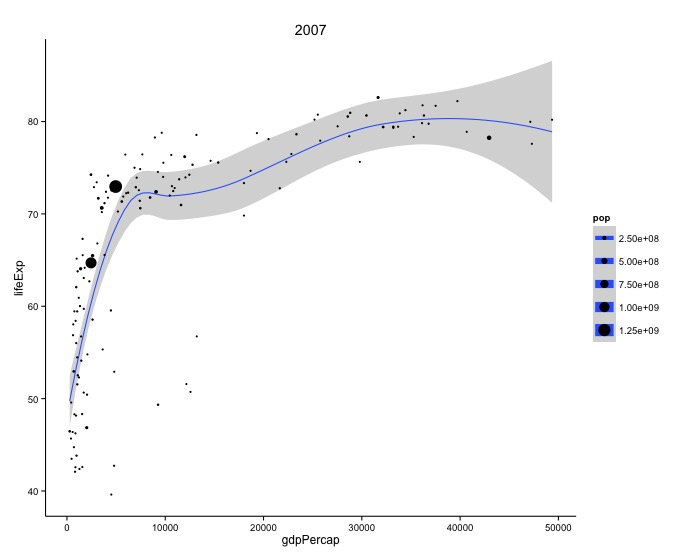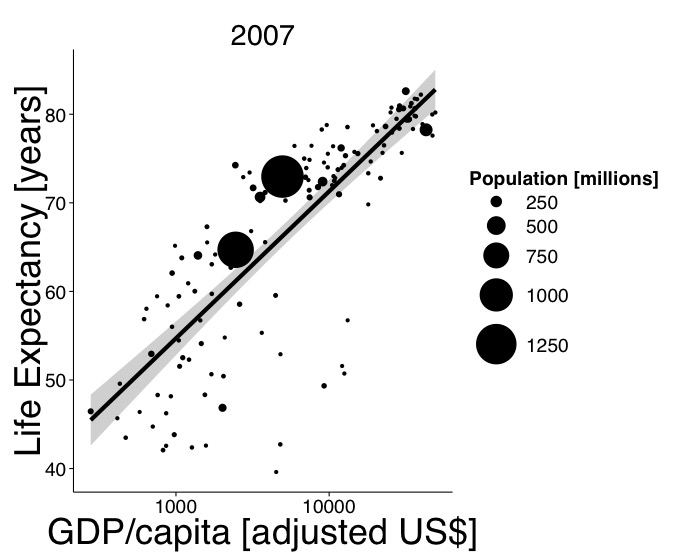# Bubble Charts

## Overview

Teaching: 10 min
Exercises: 10 min
Questions
• What kind of scientific question does a bubble chart address?

• How do I generate a bubble chart with `ggplot2`?

Objectives
• To generate a bubble chart using `ggplot2`

Lead students to walk through decision making steps from question to chart.

• “What is the relationship of GDP per capita, life expectancy, and population across the world per country in 2007?”
• Relationship with three variables (at least two are continuous)
• Bubble Chart

Implement bubble chart.

• `geom_point()`
• `geom_smooth()`
• `scale_manual()`

## Bubble Chart Sort and Fill-in Challenge

Sort and fill in the missing pieces of the following code to generate a bubble chart.

``````ggplot(data, aes(x = ________, y = ________, size = pop_2007)) +
geom_point() +
theme_classic()
geom_smooth() +
labs(x = ________, y = ________, size = "pop", title = "2007") +
``````

## Output Solution## Code Solution

``````ggplot(data, aes(x = gdpPercap_2007, y = lifeExp_2007, size = pop_2007)) +
geom_smooth() +
geom_point() +
labs(x = "gdpPercap", y = "lifeExp", size = "pop", title = "2007") +
theme_classic()
``````

## Publication Quality Bubble Chart Fill-in Challenge

Fill in the missing pieces of the following code to generate a publication quality bubble chart.

``````ggplot(data, aes(________)) +
geom_smooth(method = ________, color = "black", size = 2) +
geom_point() +
scale_size(range = c(________)) +
scale_x_log10() +
labs(x = "GDP/capita [adjusted US\$]", y = "Life Expectancy [years]",
size = "Population [millions]", title = "2007") +
theme_classic(________) +
theme(axis.title = ________)
``````

## Output Solution## Code Solution

``````ggplot(data, aes(x = gdpPercap_2007, y = lifeExp_2007,
size = pop_2007/1000000)) +
geom_smooth(method = "lm", color = "black", size = 2) +
geom_point() +
scale_size(range = c(2, 20)) +
scale_x_log10() +
labs(x = "GDP/capita [adjusted US\$]", y = "Life Expectancy [years]",
size = "Population [millions]", title = "2007") +
theme_classic(base_size = 24, base_family = "Helvetica") +
theme(axis.title = element_text(size = 36))
``````

## Key Points

• Bubble charts represent a relationship with three variables